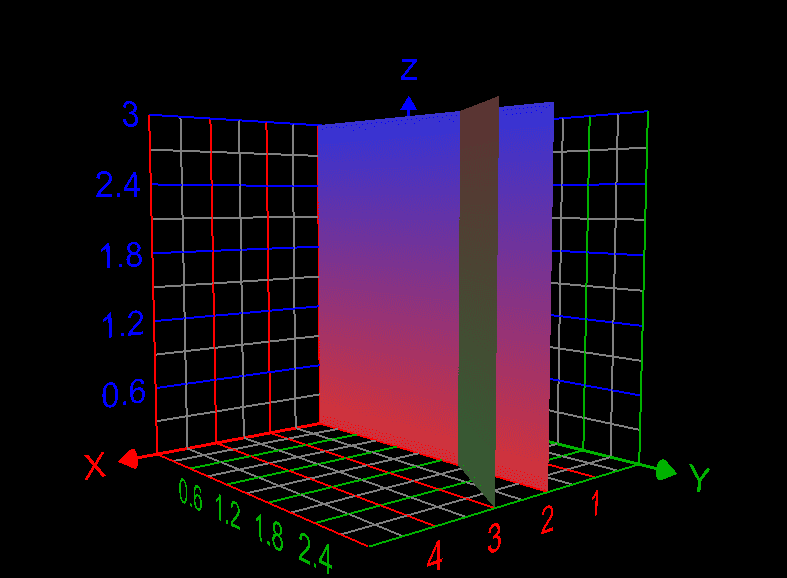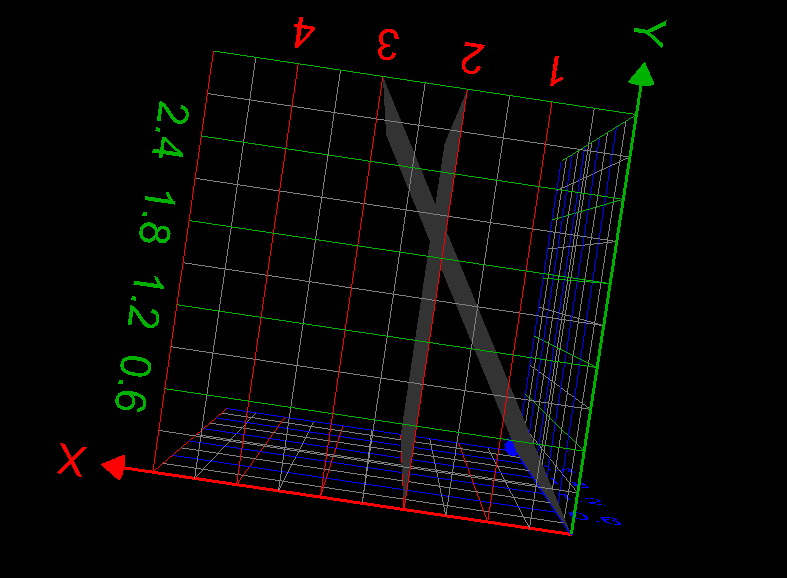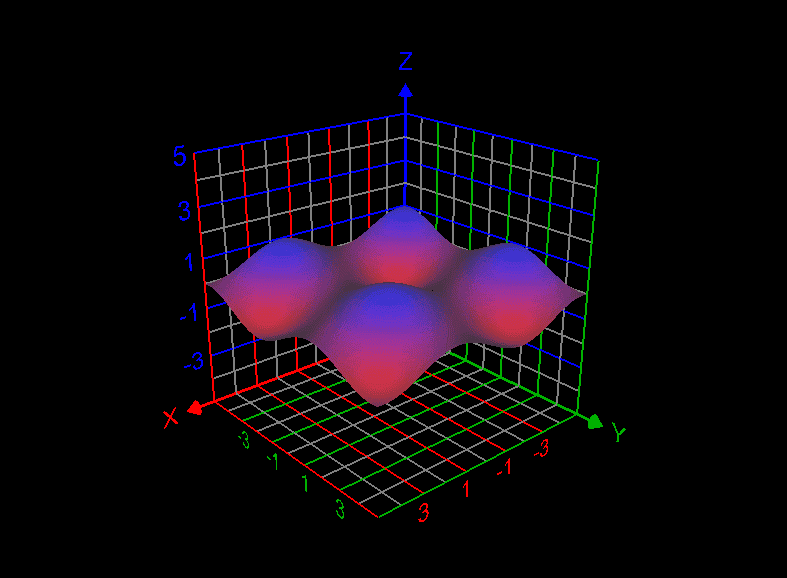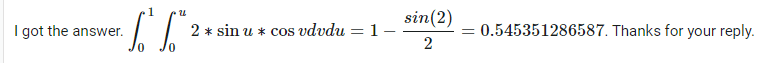# Evaluation of integral having trigonometric functions

• WMDhamnekar

#### WMDhamnekar

MHB
Homework Statement
Evaluate ## \displaystyle\iint\limits_R \sin{(\frac{x+y}{2})} \cos{(\frac{x-y}{2})}dA,## where R is the triangle with vertices (0,0), (2,0) and (1,1).
Relevant Equations
Hint: Use the change of variables ##u=\frac{x+y}{2}, v= \frac{x-y}{2}##
R is the triangle which area is enclosed by the line x=2, y=0 and y=x.
Let us try the substitution ##u = \frac{x+y}{2}, v=\frac{x-y}{2}, \rightarrow x=2u-y , y= x-2v \rightarrow x= 2u-x + 2v \therefore x= u +v##
## y=x-2v \rightarrow y=2u-y-2v, \therefore y=u- v## The sketch of triangle is as follows:But when we plot sin((x + y)/2)*cos((x-y)/2), we get the below graph:J(u,v)=|-2| = 2, So, now how to compute integration limits?

•Delta2
I computed the answer as ## \displaystyle\int_0^{0.5}\displaystyle\int_0^{1.5} 2*sin(u)*cos(v)du dv = 0.891024635191 ## . But it is wrong. Correct answer provided is ## 1- \frac{sin(2)}{2} = 0.545351286587##

How is that? What is wrong with my answer?

I computed the answer as ## \displaystyle\int_0^{0.5}\displaystyle\int_0^{1.5} 2*sin(u)*cos(v)du dv = 0.891024635191 ## . But it is wrong. Correct answer provided is ## 1- \frac{sin(2)}{2} = 0.545351286587##

How is that? What is wrong with my answer?

How did you arrive at those limits? You started with a triangle in the $(x,y)$ plane. Does it seem sensible that you would end up with a rectangle in the $(u,v)$ plane?

••WMDhamnekar and Delta2
How did you arrive at those limits? You started with a triangle in the $(x,y)$ plane. Does it seem sensible that you would end up with a rectangle in the $(u,v)$ plane?